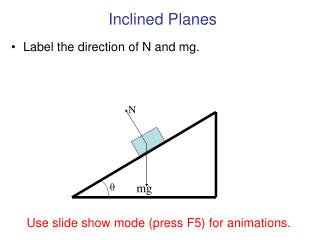# Inclined Planes - PowerPoint PPT PresentationDownload PresentationInclined Planes

Inclined Planes
Download Presentation## Inclined Planes

- - - - - - - - - - - - - - - - - - - - - - - - - - - E N D - - - - - - - - - - - - - - - - - - - - - - - - - - -
##### Presentation Transcript

1. Inclined Planes • Label the direction of N and mg. N θ mg Use slide show mode (press F5) for animations.

2. Inclined Planes • Mark the direction of acceleration a. N a θ mg

3. Inclined Planes • Choose the coordinate system with x in the same or opposite direction of acceleration and y perpendicular to x. y N x a θ mg

4. Inclined Planes • Now some trigonometry y N x a θ 90- θ mg θ

5. Inclined Planes • Replace the force of gravity with its components. y N x a mg sinθ mg cosθ θ θ mg

6. Inclined Planes • Use Newton’s second law for both the x and y directions y N x a mg sinθ mg cosθ θ θ mg The force and acceleration in the x-direction have a negative sign because they point in the negative x-direction.

7. y N x a mg sinθ mg cosθ θ θ mg Inclined Planes • Why is the component of mg along the x-axis –mgsinθ • Why is the component of mg along the y-axis –mgcosθ

8. Inclined Planes • Why is the component of mg along the x-axis: –mgsinθ • Why is the component of mg along the y-axis: –mgcosθ y N a mg sinθ x θ θ mg mg cosθ

9. θ Inclined Planes • Why is the component of mg along the x-axis: –mgsinθ • Why is the component of mg along the y-axis: –mgcosθ y N a mg sinθ x θ mg mg cosθ

10. θ Inclined Planes • Why is the component of mg along the x-axis: –mgsinθ • Why is the component of mg along the y-axis: –mgcosθ y opposite N sinθ = a hypotenuse adjacent cosθ = mg sinθ hypotenuse x θ mg mg cosθ